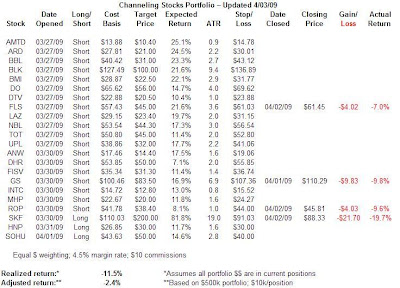## Channeling Stocks Portfolio Update

Below is the current holdings in the Channeling Stocks portfolio. This reflects the past week’s additions and subtractions. I did forget to mention that on 4/2 the Ultrashort Financial ETF, the SKF, violated its lower channel forcing a sell. My apologies.

As I mentioned in the MANDA update, please note that the realized return is based on a total portfolio value equal to exactly that of the current holdings (where each holding is of equal dollar value). If a static portfolio value is assumed, the realized return will be a different number (either more or less). There’s no one correct way to calculate the theoretical total return. I prefer the former method for a couple of reasons: it’s easier to calculate and easier to demonstrate, i.e., the reader can see exactly how each trade performed.

I decided in this portfolio to compare the dynamic realized return to a “static” return. To calculate the static return, I began with a portfolio of \$500,000 with each position set at \$10,000. (\$10 commissions and 4.5% margin interest computed monthly are also assumed.) You can see that the difference between these two returns is quite dramatic: -11.5% for the dynamic portfolio versus only -2.4% for the static one. Obviously, this is because there’s a lot of cash in the latter portfolio as opposed to none in the former which cushions the drawdown.Click on table to enlarge.